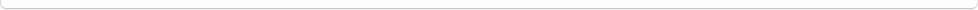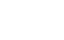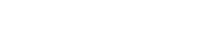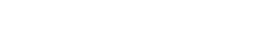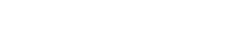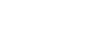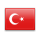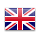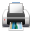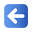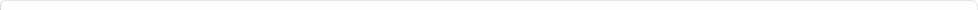Description of Individual Course Units
 Course Unit Code Course Unit Title Type of Course Unit Year of Study Semester Number of ECTS Credits 20150102001100 CALCULUS - I Compulsory 1 1 5
Level of Course Unit
First Cycle
Objectives of the Course
To give basic concepts about mathematics, to give the concepts of limit, continuity, derivative in single variable functions.
Name of Lecturer(s)
Dr.Öğr.Üyesi Hasan KARA
Learning Outcomes
 1 Defines the increasing and decreasing functions with tangent and normal equation. 2 Defines the increasing and decreasing functions with tangent and normal equation. 3 Calculates the limit of indeterminate states by using derivative. 4 Calculates the limit of indeterminate states by using derivative. 5 Defines asymptotes with maximum and minimum of functions. 6 Defines asymptotes with maximum and minimum of functions. 7 Explains the curve drawings. 8 Explains the curve drawings. 9 Solve engineering problems by using derivative. Calculates approximate using differential. 10 Solve engineering problems by using derivative. Calculates approximate using differential. 11 Define concepts of set and number sets. Explain the concepts of identity, equation and inequality. 12 Define concepts of set and number sets. Explain the concepts of identity, equation and inequality. 13 Defines the properties of functions and functions. 14 Defines the properties of functions and functions. 15 Defines trigonometric, inverse trigonometric and hyperbolic functions, Partial functions and specially defined functions (absolute value, exact value, sign functions). 16 Defines trigonometric, inverse trigonometric and hyperbolic functions, Partial functions and specially defined functions (absolute value, exact value, sign functions). 17 Explains the concept of limit and makes limit calculation with limit definition. Prove the rules used for limit calculation. 18 Explains the concept of limit and makes limit calculation with limit definition. Prove the rules used for limit calculation. 19 Defines right and left sided limits. He knows vague situations. 20 Defines right and left sided limits. He knows vague situations. 21 Defines the concept of continuity in functions and knows types of discontinuity. 22 Defines the concept of continuity in functions and knows types of discontinuity. 23 Explain the concept of derivative and make derivative calculations with the definition of derivative. Provides the rules of derivation with the definition of derivative. 24 Explain the concept of derivative and make derivative calculations with the definition of derivative. Provides the rules of derivation with the definition of derivative. 25 Defines the derivative of trigonometric and inverse trigonometric functions, exponential and logarithmic functions, hyperbolic and inverse hyperbolic functions. 26 Defines the derivative of trigonometric and inverse trigonometric functions, exponential and logarithmic functions, hyperbolic and inverse hyperbolic functions. 27 Calculates higher order derivatives. The parametric equations describe the derivatives of the given functions. Explain the derivative of closed functions. 28 Calculates higher order derivatives. The parametric equations describe the derivatives of the given functions. Explain the derivative of closed functions.
Mode of Delivery
Daytime Class
Prerequisites and co-requisities
None
Recommended Optional Programme Components
None
Course Contents
Preliminaries, Functions, Limits and Continuity, Derivatives, Applications of Derivatives.
Weekly Detailed Course Contents
 Week Theoretical Practice Laboratory 1 Sets. Number Sets. Equations. Identities. Inequalities. 2 Function concept. Function varieties (Polynomial function, rational function, exponential and logarithm function and the widest definition of these functions). 3 Function types (Trigonometric, inverse trigonometric and hyperbolic functions. Partial functions, specially defined functions (Absolute value, exact value, sign functions) 4 Limit concept and limit calculation. Proof of the rules used for limit calculation. Sandwich theorem. Limits of trigonometric functions. 5 Right and left sided limits. Uncertain cases (0/0, infinite / infinite, 0.infinite, infinite-infinite, 1 ^ infinite) 6 oncept of continuity in functions. Types of discontinuity. Properties of continuous functions (Intermediate theorem, absolute maximum and minimum, local maximum and minimum definitions) 7 The concept of derivative and derivative calculus. Derivation of derivative with the definition of derivative rules. Derivative of inverse function. 8 Midterm 9 Derivative of trigonometric and inverse trigonometric functions. Derivative of exponential and logarithm functions. Derivative of hyperbolic and inverse hyperbolic functions. 10 Higher order derivative. Derivatives of functions given parametric equations. Derivative of closed functions. 11 Tangent and normal equation. Increasing and decreasing functions 12 Indefinite cases (examination with L’Hopital Rule). 13 Maximum and minimum functions, asymptotes, Curve drawings. 14 Engineering problems. Approximate calculation with differential. 15 Engineering problems. Approximate calculation with differential. 16 Final Exam
Recommended or Required Reading
1-Matematik Analiz ve Analitik Geometri, Edwards ve Penney, Çeviri Editörü Prof.Dr. Ömer Akın 2-Genel Matematik, Prof. Dr. Mustafa Balcı Calculus, Robert Ellis-Denny Gulick 3-Genel Matematik, Prof. Dr. Ekrem Kadıoğlu, Prof. Dr. Muhammet Kamalı
Planned Learning Activities and Teaching Methods
Assessment Methods and Criteria
 Term (or Year) Learning Activities Quantity Weight Midterm Examination 1 100 SUM 100 End Of Term (or Year) Learning Activities Quantity Weight Final Examination 1 100 SUM 100 Term (or Year) Learning Activities 40 End Of Term (or Year) Learning Activities 60 SUM 100
Language of Instruction
Turkish
Work Placement(s)